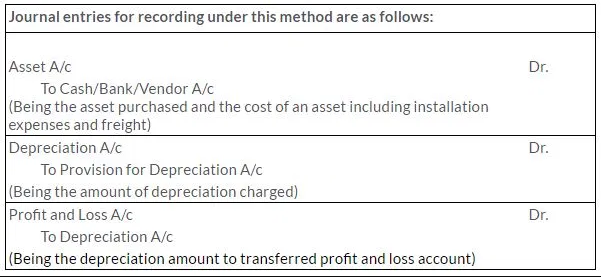# Chapter 7 – Depreciation, Provisions and Reserves Questions and Answers: NCERT Solutions for Class 11 Accountancy (Part 1)

Class 11 Accountancy (Part 1) NCERT book solutions for Chapter 7 - Depreciation, Provisions and Reserves Questions and Answers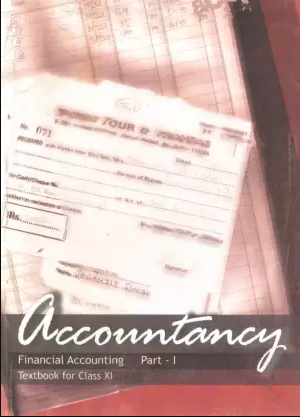## Q3. What are the causes of depreciation?

### Solution: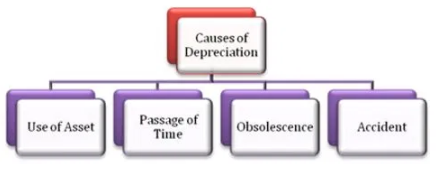## Q2. Discuss in detail the straight line method and written down value method of depreciation.Distinguish between the two and also give situations where they are useful.

### Solution: The two methods of depreciation are 1. Fixed percentage on original cost or straight line method 2. Fixed percentage on diminishing balance or written down value method Straight Line Method According to this method, a fixed and equal amount is charged as depreciation for every accounting period during the life time of an asset. This method is based on the assumption of equal usage of time over asset’s entire useful life. Hence, the amount of depreciation is same from period to period over the life of the asset. Depreciation amount can be calculated by using the following formula: If the asset has a residual value at the end of its useful life, the amount to be written of every year is as follows: Depreciation = Cost of asset – Estimated net residual value / No. of years of expected life If the annual depreciation amount is given then we can calculate the rate of depreciation as follows: Rate of depreciation = Annual depreciation amount / Cost of asset * 100 Advantages of Straight Line Method 1. Simple to calculate the depreciation amount 2. Assets can be depreciated up to the estimated scrap value 3. Easy to understand the amount of depreciation 4. Every year, the same amount of depreciation is debited to profit and loss account, and hence the effect on profit and loss account will remain the same. Disadvantages of Straight Line Method 1. Interest on capital invested in assets is not provided in this method. 2. Over the years, the work efficiency of assets decreases and repair expenses increases. Therefore, there is burden on the profit and loss account. 3. Book value of the assets becomes zero but still the assets are used in the business. Written Down Value Method In this method depreciation is charged on the book value of the asset and the amount of depreciation reduces year after year. It implies that a fixed rate on the written down value of the asset is charged as depreciation every year over the expected useful life of the asset. The rate of depreciation is applicable to the book value but not to the cost of asset. Rate of depreciation can be ascertained on the basis of cost, scrap value and useful life of the asset as follows: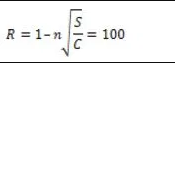## Q3.Describe in detail two methods of recording depreciation. Also give the necessary journal entries.

### Solution: The two methods of recording depreciation are as follows: 1. When Depreciation is Charged or Credited to the Assets Account In this method, depreciation is deducted from the asset value and charged (debited) to profit and loss account. Hence the asset value is reduced by the amount of depreciation.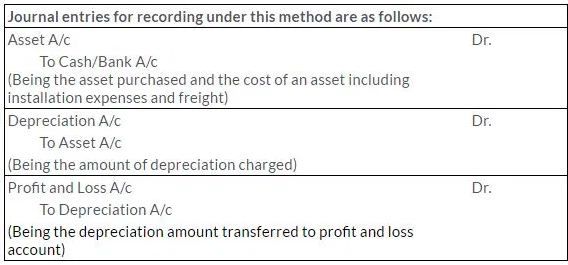### In the Balance sheet, asset appears at its written down value which is cost less depreciation charged till date. In this method, the original cost of an asset and the total amount of depreciation which has been charged cannot ascertain from this balance sheet. 2. When Depreciation is Credited to Provision for Depreciation Account In this method, depreciation is credited to the provision for depreciation account or accumulated depreciation account every year. Depreciation is accumulated in a separate account instead of adjusting into the asset account at the end of each accounting period. In the balance sheet, the asset will continue to appear at the original cost every year. Thus, the balance sheet shows the original cost of the asset and the total amount of depreciation charged on asset.